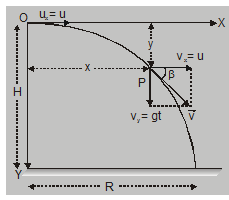Deepak Scored 45->99%ile with Bounce Back Crack Course. You can do it too!

# Horizontal Projectile motion - Motion in Plane - eSaral

Hey, do you want to learn about Horizontal projectile motion? If yes. Then you are at the right place.

## Horizontal Projectile motion

Suppose a body is thrown horizontally from point O, with velocity u. Height of O from ground = H. Let X-axis be along horizontal and Y-axis be vertically downwards and origin O is at point of projection as shown in fig. Let the particle be at P at a time t. The co-ordinates of P are (x, y) Distance travelled along X-axis at time t with uniform velocity i.e. Velocity of projection and without acceleration.The horizontal component of velocity $\mathrm{V}_{\mathrm{x}}$ = u

and horizontal displacement x = u . t….(1)

to calculate y, consider vertical motion of the projectile

initial velocity in vertical direction $\mathrm{u}_{\mathrm{y}}$ = 0.

acceleration along y direction $a_{y}$ = g (acc. due to gravity)

so

$v_{v}=a_{y} t$

(y comp. of velocity at time t)

Or

$v_{y}=g t$....(2)

(as body were dropped from a height)

Resultant velocity at time t is

$\overrightarrow{\mathrm{v}}=\mathrm{u} \hat{\mathrm{i}}+(\mathrm{gt}) \hat{\mathrm{j}}$

$v=\sqrt{u^{2}+(g t)^{2}}$

if $\beta$ is the angle of velocity with X-axis (horizontal) $\tan \beta=\frac{\mathrm{gt}}{\mathrm{u}}$

and

$y=\frac{1}{2} g t^{2}$......(3)

Or

$y=\frac{1}{2} g\left(\frac{x}{u}\right)^{2}$

[from equation (i) $\left.\mathrm{t}=\frac{\mathrm{x}}{\mathrm{u}}\right]$

Or

$y=\frac{g}{2 u^{2}} \cdot x^{2}$

Or

$y=k x^{2}$

here $\mathrm{k}=\frac{\mathrm{g}}{2 \mathrm{u}^{2}} \quad(\mathrm{k}$ is constant)

This is eqn. of a parabola.

A body thrown horizontally from a certain height above the ground follows a parabolic trajectory till it hits the ground.
1. Time of flight$\mathrm{T}=\sqrt{\frac{2 \mathrm{H}}{\mathrm{g}}}$ [as $\left.\mathrm{y}=\frac{1}{2} \mathrm{gt}^{2}, \mathrm{~T}=\sqrt{\frac{2 \mathrm{H}}{\mathrm{g}}}\right]$

2. Range horizontal distance covered = R.R = u × time of flight$\mathrm{R}=\mathrm{u} \cdot \sqrt{\frac{2 \mathrm{H}}{\mathrm{g}}}$

$\left[\because \mathrm{H}=\frac{\mathrm{g}}{2 \mathrm{u}^{2}} \mathrm{R}^{2}\right]$

3. Velocity when it hits the ground$\mathrm{v}_{\mathrm{g}}=\sqrt{\mathrm{u}^{2}+2 \mathrm{gH}}$